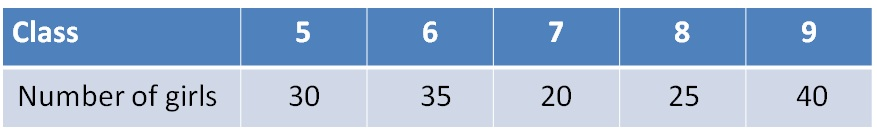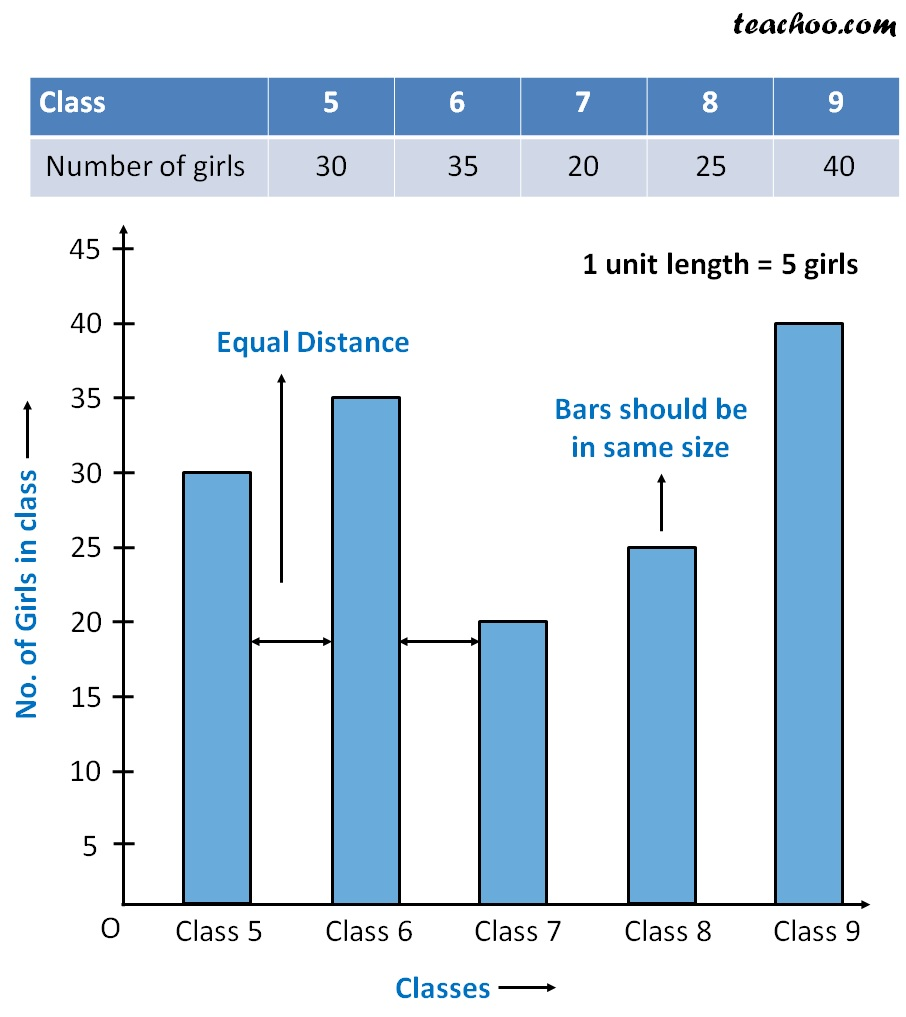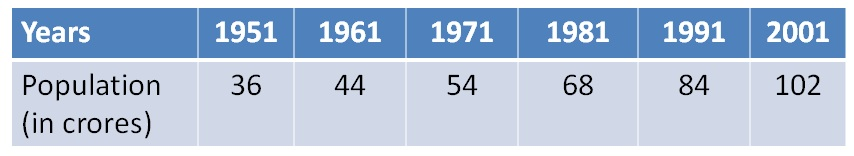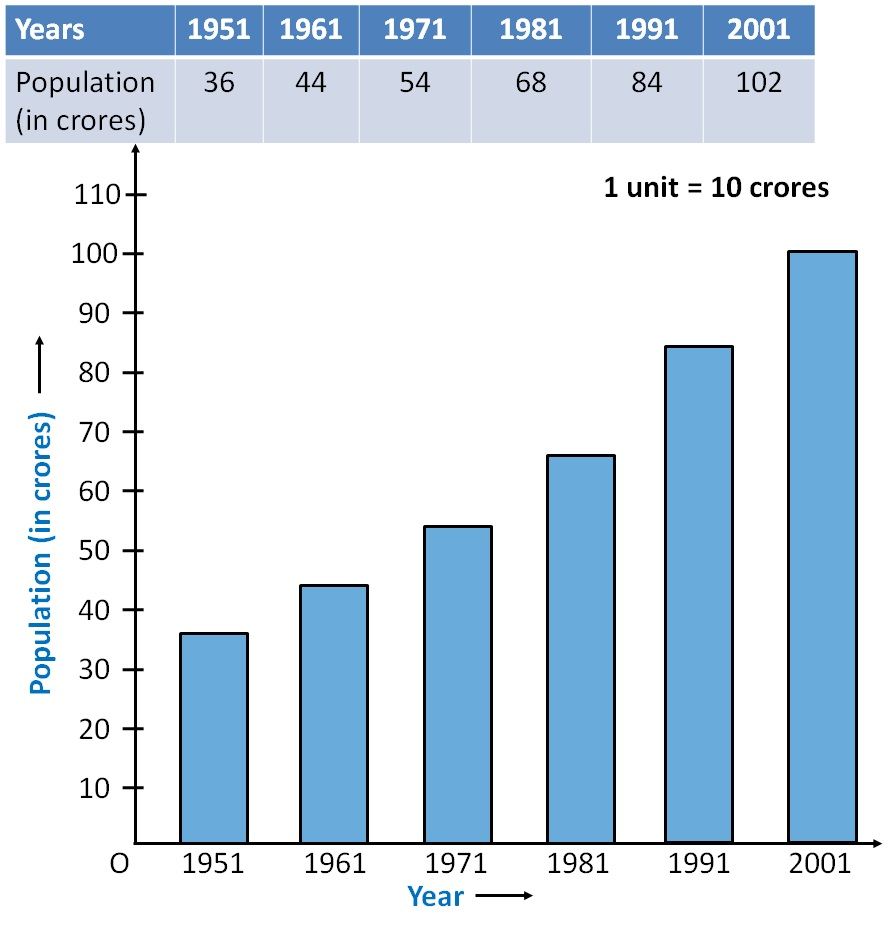Suppose we have data of

Number of girls in class 5 to 9We draw a bar graph like thisWe also take another example

Population of India from 1951 to 2001Since we have to go from 36 to 102

We draw graph from 0 to 110 with gaps of 101. Chapter 9 Class 6 Data Handling
2. Concept wise
3. Bar Graph

Transcript

Bar Graph Suppose we have data of Number of girls in class 5 to 9 We draw a bar graph like this We also take another example Population of India from 1951 to 2001 Since we have to go from 36 to 102 We draw graph from 0 to 110 with gaps of 10

Bar Graph